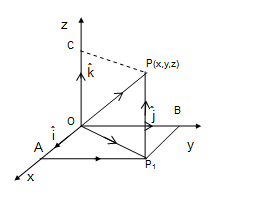Q

# Give answer! - Vector Algebra - JEE Main

The area (in sq. units) of the parallelogram whose diagonals are along the vectors• Option 1)

26

• Option 2)

65

• Option 3)

20

• Option 4)

52

115 Views

As we learnt in

Vector Product of two vectors(cross product) -

If  and  are two vectors and  is the angle between them , then

- wherein

is unit vector perpendicular to both

and

Position vector of a point -

- whereinArea of parallelogram =

where  are diagonals

magnitude

Option 1)

26

This option is incorrect.

Option 2)

65

This option is correct.

Option 3)

20

This option is incorrect.

Option 4)

52

This option is incorrect.

Exams
Articles
Questions